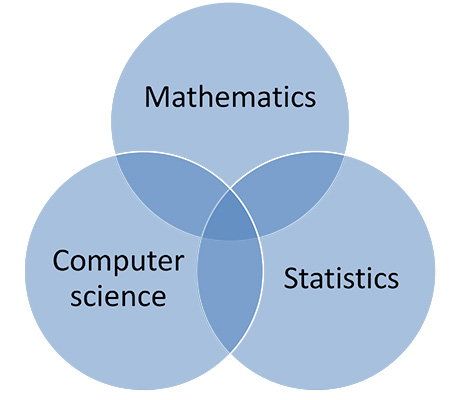# Employees working on the subject of mathematics

DTU Compute consists of 10 research sections, all working in a combination of Mathematics, Statistics, Computer Science.

 Image analysis, mathematical modeling, statistics Anders Bjorholm Dahl Mathematical modeling, probability, applied probability Anders Stockmarr Digital shape modeling, digital shape representation, interactive 3D modeling, real-time rendering, computer graphics Andreas Bærentzen Mathematics, graph theory, combinatorics Carsten Thomassen Analysis of algorithms, complexity theory, randomized search heuristics, evolutionary algorithms Carsten Witt Dynamical systems, iteration, mathematical analysis, applied mathematics, numerical analysis Christian Henriksen Discrete mathematics, mathematical logic Flemming Nielson Algorithms, data structures, compression, approximation algorithms, graph algorithms Inge Li Gørtz Functional analysis, Harmonic analysis, Frame theory, Gabor analysis, Wavelet theory Jakob Lemvig Differential geometry, Geometric design, Shape optimization, Isogeometric analysis, Applied Mathematics, Mathematics in industry, Geometrical effects in physics Jens Gravesen Computer graphics, digital image synthesis, realistic rendering, material appearance, light scattering Jeppe Revall Frisvad Discrete Mathematics, Algebraic Coding Theory, Computer Algebra Johan Rosenkilde Model Predictive Control, Numerical optimization, Scientific Computing, Numerical solution of differential equations, Process control John Bagterp Jørgensen Logic, Foundations of mathematics, Meta-Logic, Proof assistants, Type theory and higher-order logic Jørgen Villadsen Applied mathematics, Inverse problems, Partial differential equations, Tomography, Scientific Computing Kim Knudsen Cryptology, IT-security, Cyber security, Digital signatures, Encryption, authentication Christian D. Jensen Industrial mathematics, nonlinear dynamics, nonlinear partial and ordinary differential equations, solitons, chaos Mads Peter Sørensen Optimization, numerical methods, signals & systems, inverse problems, machine learning Martin Skovgaard Andersen Functional Analysis, Partial Differential Equations, Pseudodifferential Operators, Control for PDE's Michael Pedersen Dynamical systems Morten Brøns Functional Analysis, Harmonic Analysis, Frame Theory, Gabor Analysis, Wavelet Theory Ole Christensen Inverse problems, Tomographic reconstruction algorithms, Matrix computations, Numerical analysis Per Christian Hansen Applied algebra, Algebraic curves, Error-correcting codes, Discrete mathematics Peter Beelen Differentail geometry, Knot theory, Computational structural biology, Protein structure comparison and prediction, Peter Røgen Algorithms, data structures, compression Philip Bille Applied mathematics, Industrial modelling, Ordinary differential equations, Analytical mechanics Poul G. Hjorth Medical Image Analysis, 3D shape analysis, Computational Geometry, 3D surface scanning Rasmus R. Paulsen Mathematical logic Sebastian Mödersheim Differential geometry, global geometric analysis, learning of mathematics Steen Markvorsen

## ContactChristian Henriksen
Associate Professor
DTU Compute
+45 45 25 30 54

## ContactJakob Lemvig
Associate Professor
DTU Compute
+45 45 25 30 65

## ContactJens Gravesen
Associate Professor
DTU Compute
+45 45 25 30 64

## ContactMorten Brøns
DTU Compute
+45 45 25 30 67

## ContactOle Christensen
Professor
DTU Compute
+45 45 25 30 43

## ContactPeter Beelen
Professor
DTU Compute
+45 45 25 30 22

## ContactSteen Markvorsen
Professor
DTU Compute
+45 45 25 30 49

## ContactCarsten Thomassen
Professor
DTU Compute
+45 45 25 30 58
https://www.compute.dtu.dk/english/basic-scientific-areas/mathematics
31 MARCH 2023